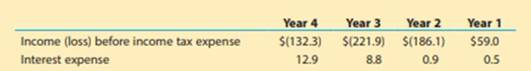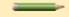# Analyze Aeropostale Aeropostale, Inc. (ARO) is a specially fashion retailer targeting young adults. The income before income tax expense and interest expense for four recent years follow (in millions): a. Compute the times interest earned ratio for each year. Round to one decimal place. b. Plot the four points on a graph with the year numbers on the horizontal axis, beginning with Year 1. c. Interpret the trend in the ratio from your graph. d. What happened to interest expense in Year 4? What might be the cause?### Corporate Financial Accounting

15th Edition
Carl Warren + 1 other
Publisher: Cengage Learning
ISBN: 9781337398169

#### Solutions

Chapter
Section### Corporate Financial Accounting

15th Edition
Carl Warren + 1 other
Publisher: Cengage Learning
ISBN: 9781337398169
Textbook Problem
1 views

## Analyze AeropostaleAeropostale, Inc. (ARO) is a specially fashion retailer targeting young adults. The income before income tax expense and interest expense for four recent years follow (in millions):a. Compute the times interest earned ratio for each year. Round to one decimal place.b. Plot the four points on a graph with the year numbers on the horizontal axis, beginning with Year 1.c.Interpret the trend in the ratio from your graph.d.What happened to interest expense in Year 4? What might be the cause?

a.

To determine

To Compute: Times-interest-earned ratio for each year.

### Explanation of Solution

Times-Interest-Earned ratio: It is the ratio that quantifies a business ability to pay interest expense. It is calculated as shown below:

Times-interest-earned ratio}=Incomebeforeincometax+Interest expenseInterest expense

Determine the times-interest-earned ratio for each year.

Year 4:

Times-interest- earned ratio}=Income(Loss)beforeincometaxes+Interest expenseInterest expense=($132.3)+$12.9$12.9=($119.4)$12.9=(9.3) Year 3: Times-interest- earned ratio}=Income(Loss)beforeincometaxes+Interest expenseInterest expense=($221.9)+\$8

b.

To determine

To plot: the graph with the four points and the years in the horizontal axis.

c.

To determine

To interpret: the trend in the ratio from the graph.

d.

To determine

To Explain: the reason for the significant increase in the interest expense.

### Still sussing out bartleby?

Check out a sample textbook solution.

See a sample solution

#### The Solution to Your Study Problems

Bartleby provides explanations to thousands of textbook problems written by our experts, many with advanced degrees!

Get Started

Find more solutions based on key concepts
How are inflation and unemployment related in the short run?

Principles of Economics (MindTap Course List)

List seven reasons risk management might increase the value of a firm.

Fundamentals of Financial Management (MindTap Course List)

Briefly explain what is meant by the term efficiency continuum.

Fundamentals Of Financial Management, Concise Edition (mindtap Course List)

A sinking fund can be set up in one of two ways: The corporation makes annual payments to the trustee, who inve...

Fundamentals of Financial Management, Concise Edition (with Thomson ONE - Business School Edition, 1 term (6 months) Printed Access Card) (MindTap Course List)

Could the United States ever experience another Great Depression? Why or why not?

Macroeconomics: Private and Public Choice (MindTap Course List)